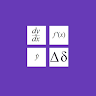# Calculus Formulas

## Information

 Package com.binarytuts.calculusformulas Version 1.0.2 (3) Updated 2019-07-29 Size 8 MB Installs 1,000 Category Education

## Description

Calculus is involves in the study of ‘continuous change,’ and their application to solving equations. It has two major branches:

1: Differential Calculus that is concerning rates of change and slopes of curves.
2: Integral Calculus concerning accumulation of quantities and the areas under and between curves.

Both Differential Calculus and Integral Calculus make use of the fundamental notions of convergence of infinite sequences and infinite series to a well-defined limit. These two branches are related to each other by the fundamental theorem of calculus

The Differential Calculus splits up an area into small parts to calculate the rate of change. While, the Integral calculus joins small parts to calculates the area or volume. In short, it is the method of reasoning or calculation.

In this app you can see a list of Calculus Formulas such as integral formula, derivative formula, limits formula etc.

Limits Formulas contains:
Limits Definitions.
Relationship between the limit and one-sided limits.
Limits Properties Formulas.
Basic Limit Evaluations Formulas.
Evaluation Techniques Formulas.
Some Continuous Functions.
Intermediate Value Theorem.
Solve Any Calculus Limit.

Derivatives Formulas contains:
Derivatives Definition and Notation.
Interpretation of the Derivative.
Basic Properties and Formulas.
Common Derivatives.
Chain Rule Variants.
Higher Order Derivatives.
Implicit Differentiation.
Increasing/Decreasing – Concave Up/Concave Down.
Extrema.
Mean Value Theorem.
Newton’s Method.
Related Rates.
Optimisation .

Integrals Formulas contains:

Integrals Definitions.
Fundamental Theorem of Calculus.
Properties.
Common Integrals.
Standard Integration Techniques.
Improper Integral.
Approximating Definite Integrals.

Very handy app for students of Mathematics.